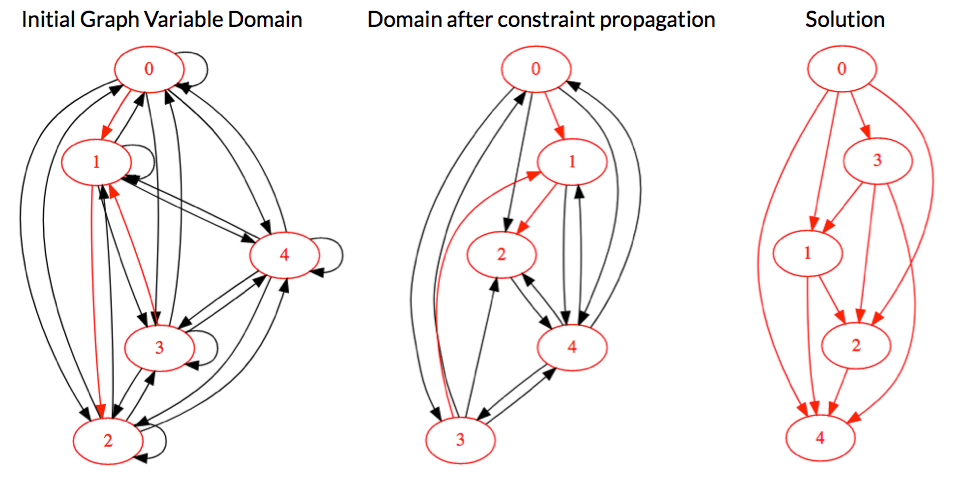# Choco Solver

### Using Graph Variables within Choco Solver

In this example, we model and solve the Directed Acyclic Graph (DAG) Problem, which consists in finding a directed graph without circuit, using Constraint Programming. For this purpose, we use the Graph extension of Choco Solver in order to create a graph variable and graph constraints. Note that since Choco Graph 4.2.0, graph variable domain may be exported in the Graphviz format.
``````/*
* Copyright (C) 2017 COSLING S.A.S.
*
* you may not use this file except in compliance with the License.
* You may obtain a copy of the License at
*
*
* Unless required by applicable law or agreed to in writing, software
* WITHOUT WARRANTIES OR CONDITIONS OF ANY KIND, either express or implied.
* See the License for the specific language governing permissions and
*/

import org.chocosolver.graphsolver.GraphModel;
import org.chocosolver.graphsolver.variables.DirectedGraphVar;
import org.chocosolver.solver.variables.IntVar;
import org.chocosolver.util.objects.graphs.DirectedGraph;
import org.chocosolver.util.objects.setDataStructures.SetType;

/**
* Example of Graph Variable in Choco Solver: computes a directed acyclic graph
* @author Jean-Guillaume FAGES (cosling)
* @version choco-solver-4.0.4
* @version choco-graph-4.2.0
*/
public class DAG {

public static void main(String[] args){
int n = 5;
GraphModel model = new GraphModel();

// VARIABLE COUNTING THE NUMBER OF ARCS
IntVar nbArcs = model.intVar("arcCount", 0, n * n, true);

// GRAPH VARIABLE : initial domain (every node belongs to the solution)
DirectedGraph GLB = new DirectedGraph(model, n, SetType.BITSET, true);
DirectedGraph GUB = new DirectedGraph(model, n, SetType.BITSET, true);
GLB.addArc(0,1); // some arbitrary mandatory arcs
for (int i = 0; i < n; i++) {
for (int j = 0; j < n; j++) {
}
}

DirectedGraphVar dag = model.digraphVar("dag", GLB, GUB);

// CONSTRAINTS
model.noCircuit(dag).post();
model.nbArcs(dag, nbArcs).post();

// SOLVING AND PRINTS
System.out.println(dag.graphVizExport()); // displays initial graph domain
try {
model.getSolver().propagate(); // propagates constraints (without branching)
e.printStackTrace();
}
System.out.println(dag.graphVizExport()); // displays graph domain after propagation
if (model.getSolver().solve()){
System.out.println("solution found : "+nbArcs);
System.out.println(dag.graphVizExport()); // displays solution graph
}
model.getSolver().printStatistics();
}
}
```
```

The domain of the graph variable before and after constraint propagation, as well as in the first solution found is displayed below thanks to webgraphviz: